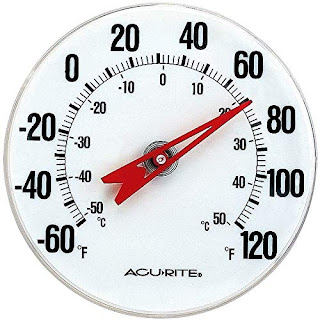An analog computer is used to process analog data. Used in calculation of physical variables such as voltage, pressure, temperature, speed etc. Analog computer performs calculations with the help of measures. Used for scientific and engineering applications.Examples of analog computers:

• Analog thermometer: used to measure temperature.
• Analog speedometer: Where the position of the needle on dial represents the speed of the car.
• Seismograph: Used to detect and record Earthquakes